# Abstract

The analytical solutions for the natural frequencies and mode shapes of the rectangular plate on foundation with four edges free is presented by using the finite cosine integral transform method. In the analysis procedure, the classical Kirchhoff rectangular plate is considered and the foundation is modelled as the Winkler elastic foundation. Because only are the basic dynamic elasticity equations of the thin plate on elastic foundation adopted, it is not need prior to select the deformation function arbitrarily. Therefore, the solution developed by present paper is reasonable and theoretical. In order to illuminate the correction of formulations, the numerical results are also presented to comparing with that of the other references.

Elastic foundation; Rectangular plate with four edges free; natural frequencies Vibration mode shapes; Finite cosine integral transform

Vibration of plate on foundation with four edges free by finite cosine integral transform method

Yang Zhong* * Author email: zhongy@dlut.edu.cn. ; Xue-feng Zhao; Heng Liu

Department of Civil Engineering Dalian University of Technology P.R.China

ABSTRACT

The analytical solutions for the natural frequencies and mode shapes of the rectangular plate on foundation with four edges free is presented by using the finite cosine integral transform method. In the analysis procedure, the classical Kirchhoff rectangular plate is considered and the foundation is modelled as the Winkler elastic foundation. Because only are the basic dynamic elasticity equations of the thin plate on elastic foundation adopted, it is not need prior to select the deformation function arbitrarily. Therefore, the solution developed by present paper is reasonable and theoretical. In order to illuminate the correction of formulations, the numerical results are also presented to comparing with that of the other references.

Keywords: Elastic foundation, Rectangular plate with four edges free, natural frequencies Vibration mode shapes, Finite cosine integral transform

1 INTRODUCTION

The vibrations of rectangular thin plates with various boundary conditions are of much importance in all the fields of civil, mechanical, and aerospace engineering. To conduct an accurate free vibration analysis of rectangular plates is necessary for controlling the resonance thus ensuring the safety of plates. Actually, the vibrations of rectangular thin plates have been extensively investigated for many years. The related publications can be counted in thousands, like in Leissa (1993). A literature survey reveals that most previous investigations have dealt with a scheme or technique that is only suitable for a particular type of boundary condition. Due to the mathematical complexity of the situation, it is well known that the analytical solutions are generally available only for plates that are simply supported along at least one pair of opposite edges. Leissa gave a survey of research on rectangular plate problems up to 1970, like in Warburton (1954) and Leissa (1973). The further overview up to the beginning of this century is presented in Warburton and Warburton & Edney (1984). One of the most commonly used methods in free vibration analysis of plates is the Rayleigh-Ritz energy technique, where appropriate functions associated with various boundary conditions are chosen to describe the lateral deflection of the deformed plates. The chosen functions normally do not satisfy both the governing differential equations and boundary conditions. Gorman used the superposition technique to solve approximately free vibration problems of plates for various geometries and boundary conditions, like in Gorman and Gorman (1982). A set of static beam functions was used to determine the natural frequencies of elastically restrained plates, like in Bapat et al (1988) and Zhou (1996). Hurlebaus et al. (2001) have extended the Fourier series solution to the problems with more complicated boundary conditions than the simply supported one. The other numerical approaches such as the finite element method as in Yang (1972) and boundary element method as in Zafrang (1995) were usually adopted by many researchers to analyze the plate on elastic foundation.

Integral transform is one of the effective approaches to obtain the analytical solutions of some partial differential equations used in elasticity, like in Sneddon (1972). This method has often been utilized to analyze some structural engineering problems, like in Sneddon (1981). However, based on the author's knowledge, there are no reports on using the finite integral transform to analyze the rectangular plate on elastic foundation, like in Zhong et al (2009), Li et al (2009), Li et al (2011) and Li et al (2013).

In this paper, the double finite cosine integral transform method is adopted to acquire the theoretical solutions of eigenfrequncies and vibration modes for the rectangular thin plate on foundation with four edges free. In the analysis the elastic foundation was modeled by the Winkler elastic foundation. Because it only uses the basic dynamic elasticity equations of the thin plate on elastic foundation and there is no need to select the deformation function arbitrarily, the developed solution is reasonable. In order to proof the correction of formulations, the numerical results are presented to compare with those from other references.

2 VIBRATION OF PLATE ON FOUNDATION AND INTEGRAL TRANSFORM

According to the theory the classical Kirchhoff plate, the governing equation of motion for an unloaded plate on the foundation iswhere D = Eh3 / 12(1 - v2) is the flexural rigidity of plate. In which E is Young's moduli. Also is Poisson's ratios. h and ρ are the thickness and the density of plate. w(x, y, t) is the out-ofplane displacement and k is the reaction coefficient of foundation. Assuming a harmonic vibration, one way writewhere W(X, y) is the shape function describing the modes of the vibration and ω is the natural circular frequency of the plate. Substitution of Eg.(2) into Eg.(1) giveswhere λ =In order to solve the partial differential equation (3), the double finite cosine integral transform approach is exploited.

If f (x, y) is a function of the two independent variables x and y, defined on the square 0 < x < a, 0 < y < b, the definition of double finite cosine integral transform is presented by the equationThe inversion formula can be derived aswhere am = mπ / a and βn = nπ / b . a and b are the length and the width of the plate respectively.

The double integral transform of the first partial derivative term appeared in Eg. (3) may readily be written as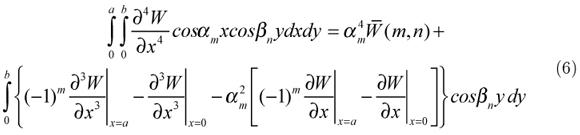and the third term can be written asThe second term is split into two parts. The first part considers the partial derivative with respect to y firstwhile the second part considers the partial derivative with respect to x firstSubstitution of equations (6-9) into equation (3) leads to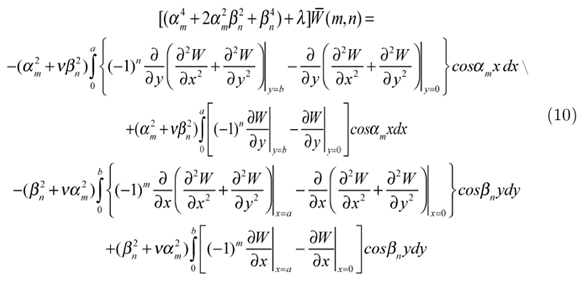The boundary conditions of a free plate areOf cause, there is another simplified expression of the boundary conditions for a free plate. Substituting the boundary conditions that are described by equation (11) and equation(14) into equation(10), one can obtain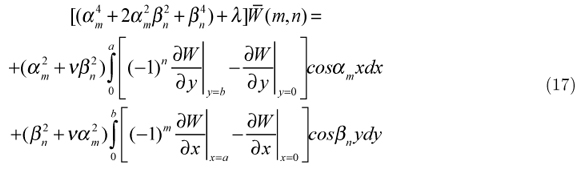Because the right-hand side of the equation (17) is definite integral, it is the constant. LetTherefore, equation (17) can be written asSubstitution of equation (18) into equation (5) gives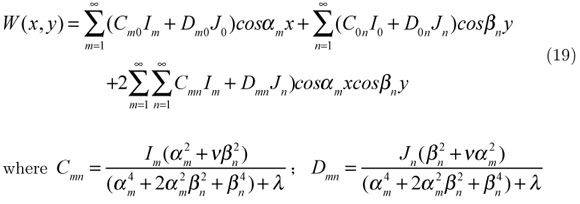where Cmn =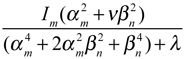; Dmn =It is clear that the equation (19) can meet the boundary conditions described by equations (11) ,(13) ,(14) and (16). From the remaining boundary conditions presented by equations (12) and (15), one can obtainEquations (20) and equation (21) make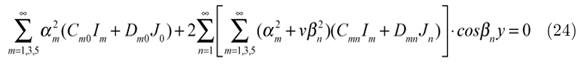Similarly, equations (23) and (24) makeEach coefficient of the cosαmx and cosβny has to be vanish. What follows is a system of homogeneous algebraic equationsEqs. (26) forms the system of four groups of an infinitely large number of linear equations in terms of the unknowns Im, Jn, I0 and J0. Non-trivial solution of those equations requires the coefficient matrix to vanish to any desired degree of accuracy. Non-trivial solution of Eqs. (26) requires the coefficient matrix to vanish. From this determinant the eigenfrequencies of the plate are calculated. The associated vibration modes are given by equation (19) after inserting the eigenfrequencies. The infinite series that occur in the corresponding equations (see Eqs. (19) and (26)) have been evaluated without any truncation using MATLAB; this has been done by specifying the upper limit of the summation index as infinity. The evaluation is exact since corresponding closed-form equivalents are automatically substituted in MATLAB.

3 NUMERICAL RESULTS

The first page includes the title of the paper, the names of the authors and their institutions, and an abstract. The following vertical spacing must be used: The previously derived expressions are applied to a rectangular thin plate on the foundation with four edges free. The geometrical and material properties are given as a = b = 4.0 m, v = 0.15, E = 3.0 × 104 MPa, h = 0.2 m, k = 5.5 × 107 N/m3 and ρ = 1750 kg/m3

In order to make the comparison with other method, the eigenfrequencies and the vibration modes are computed by the superposition method and by the present approach. The calculation results are shown in Table 1. It is obvious that the results by two different methods are in excellent agreements. This also validates the present approach is correct. Fig. 1 - 4 illuminates the corresponding vibration modes respectively.

4 CONCLUSIONS

The present paper shows that the eigenfrequencies and the vibration modes of a classical Kirch- hoff rectangular plate on foundation with four edges free can be calculated by the integral transform method. The approach is based on the finite cosine integral transform for the classical thin plate equation. One of the advantages of the method is that the results converge quickly and can be calculated with desired accuracy. The other one is that after finishing the analytical derivation for the frequency determinant the calculation of eigenfrequencies and the vibration modes for given material data and geometry becomes straightforward. The present method is convenient for sensitivity studies. As the analytical solutions for the computation of eigenfrequencies and the vibration modes of a thin plate on the foundation with four edges free are of paramount importance for many applications such as in the design of building foundations and the rigid pavements of highway and airport, the present approach of analysis provides an efficient procedure for accurate results which should be of academic and practical importance.

In revised form 20 Jun 2013

• Bapat, A. V., Venkatramani, N., Suryanarayan, S., (1988), Simulation of classical edge conditions by finite elastic restraints in the vibration analysis of plates, Journal of Sound and Vibration 120: 127-140.
• Gorman, D.J., (1980), A comprehensive study of the free vibration of rectangular plates resting on symmetrically distributed uniform elastic edge supports, Journal of Applied Mechanics 56: 893-899.
• Gorman, D.J., (1982), Free Vibration analysis of rectangular plates Elsevier North Holland, Inc.
• Hurlebaus, S. L., Gaul, J., Wang, T. S., (2001), An exact series solution for calculating the natural frequencies of orthotropic plates with completely free boundary, Journal of Sound and Vibration 244: 747-759.
• Leissa, A.W., (1993), Vibration of Plates, Acoustical Society of America.
• Leissa, A.W., (1973), The free vibrations of rectangular plates, Journal of Sound and Vibration 31: 257-293.
• Li, R., Zhong, Y., Tian, B., Liu, Y.M., (2009), On the finite integral transform method for exact bending solutions of fully clamped orthotropic rectangular thin plates. Applied Mathematics Letters 22: 1821-1827.
• Li, R., Zhong, Y., Tian, B., (2011), On new symplectic superposition method for exact bending solutions of rectangular cantilever thin plates. Mechanics Research Communications 38: 111-116.
• Li, R., Zhong, Y., Li, M.L., (2013), Analytic bending solutions of free rectangular thin plates resting on elastic foundations by a new symplectic superposition method. Proceedings of the Royal Society A 46: 468-474
• Sneddon, Ian. H., (1972), The use of integral transforms McGraw-Hill, Inc.
• Sneddon, Ian. H., (1981), The application of integral transform in elasticity McGraw-Hill, Inc.
• Warburton, G.B., (1954), The vibrations of rectangular plates, Proceeding of the Institute of Mechanical Engineers, Series A 168: 371-384.
• Warburton, G.B., (1979), Response using the Rayleigh-Ritz method, Journal of Earthquake Engineering and Structural Dynamics 7: 327-334.
• Warburton, G.B., Edney, S.L., (1984), Vibrations of rectangular plates with elastically restrained edges, Journal of Sound and Vibration 95: 537-552.
• Yang, T.Y., (1972), A finite element analysis of plate on two parameters foundation model, Computer and Structure 2: 573-616
• Zafrang, A.E., (1995), A new fundamental solution for boundary element analysis of thick plate on Winkle foundation, Int. J. Num. Eng. 38: 887-903
• Zhong, Y., Li, R., Liu, Y.M., Tian, B., (2009), On new symplectic approach for exact bending solutions of moderately thick rectangular plates with two opposite edges simply supported. International Journal of Solids and Structures 46: 2506-2513.
• Zhou, D., (1996), Natural frequencies of rectangular plates using a set of static beam functions in the Rayleigh-Ritz method, Journal of Sound and Vibration 189: 81-88.
• *
Author email:

# Publication Dates

• Publication in this collection
03 Feb 2014
• Date of issue
Oct 2014

• Accepted
20 June 2013